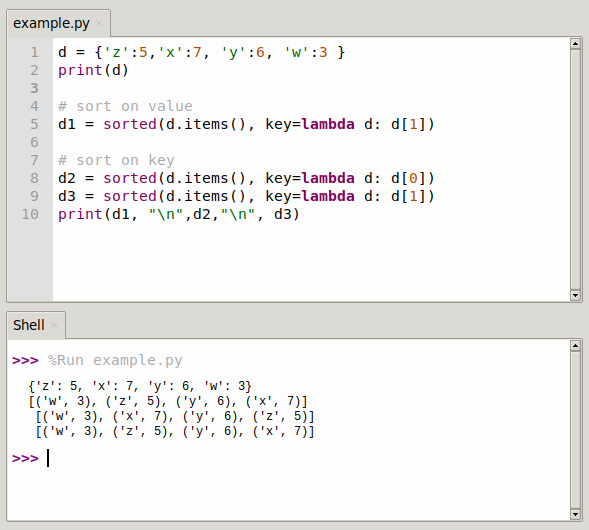# Sort a dictionary by value in Python

Python’s built-in dictionary data types are unordered, and we use key to get the corresponding value, but sometimes we need to sort the output of the item in the dictionary, either by key or by value.

Exactly how many ways are there to achieve sorted output of the dictionary content? Some wonderful solutions have been extracted below.

``>>> d = {'z':5,'x':7, 'y':6, 'w':3 }``

Then sort on key or value

``````# sort on value
>>> sorted(d.items(), key=lambda d: d)
[('w', 3), ('z', 5), ('y', 6), ('x', 7)]

# sort on key
>>> sorted(d.items(), key=lambda d: d)
[('w', 3), ('x', 7), ('y', 6), ('z', 5)]``````

## Sort dictionary by value

The program below sorts by value, you can do that either in normal or in reverse order.
We use the `sorted()` method.

``sorted(dict1.items(), key=lambda d: d)``

From large to small: plus parameter reverse

For example.

``sorted(dict1.items(), key=lambda d: d, reverse=True)``

So you can sort a dicionary by value like this:

``````>>> sorted(d.items(), key=lambda d: d)
[('w', 3), ('z', 5), ('y', 6), ('x', 7)]

>>> sorted(d.items(), key=lambda d: d, reverse=True)
[('x', 7), ('y', 6), ('z', 5), ('w', 3)]``````## Sort dictionary by key

You can sort a dictionary by key using:

``print sorted(dict1.items(), key=lambda d: d)``

Example:

``````>>> d = {'z':5,'x':7, 'y':6, 'w':3 }

# sort on key
>>> sorted(d.items(), key=lambda d: d)
[('w', 3), ('x', 7), ('y', 6), ('z', 5)]``````

## Sorting dictionary

You can easily retrieve the keys and values in Python:

``````>>> d.keys()
dict_keys(['z', 'x', 'y', 'w'])

>>> d.values()
dict_values([5, 7, 6, 3])``````

In Python 2, you were able to do this, but that no longer works:

``````import operator
x = {1: 2, 3: 4, 4:3, 2:1, 0:0}
sorted_x = sorted(x.iteritems(), key=operator.itemgetter(1))``````

d:Iterate through the list of return key pairs from dict1.items()

It would throw this exception:

``````>>> sorted_x = sorted(x.iteritems(), key=operator.itemgetter(1))
Traceback (most recent call last):
File "<stdin>", line 1, in <module>
AttributeError: 'dict' object has no attribute 'iteritems'``````

In Python 3, you can do this instead:

``````>>> import operator
>>> x = {1: 2, 3: 4, 4:3, 2:1, 0:0}
>>> sorted_x = sorted(x.items(), key=operator.itemgetter(1))
>>> print(sorted_x)
[(0, 0), (2, 1), (1, 2), (4, 3), (3, 4)]``````